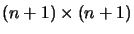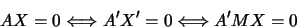Next: Linear Algebra and Homogeneous Up: Hyperplanes and Duality Previous: Desargues Theorem

### Hyperplane Transformations

In a projective space, a collineation can be defined by itsmatrix M with respect to some fixed basis. If X and X' are the coordinate vectors of the original and transformed points, we have

X' = MX.

This maps hyperplanes of points to transformed hyperplanes, and we would like to express this as transformation of dual (hyperplane) coordinates. Let A and A' be the original and transformed hyperplane coordinates. For all points X we have:The correct transformation is therefore A'M = A or:

A' = A M-1

or if we choose to represent hyperplanes by column vectors:

A't = (M-1)t At

Of course, all of this is only defined up to a scaling factor. The matrix M* = (M-1)t is sometimes called the dual of M.

Bill Triggs
1998-11-13